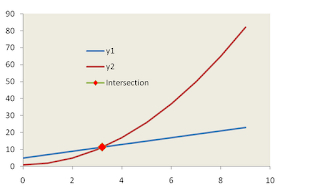# Excel : Intersection between curve and straight line

In this tutorial we will explain how to find intersection between curve and straight line.

To find intersection of curve and a straight line we first need to know the mathematical condition behind it. When two lines cross:

1. Before intersection, value of y1 is less than y2 at given value of x
2. After intersection, value of y1 is less than y2 at next level of x value (xi+1)

I consider two equations : y1=2x+5 and y2=x^2+1 . On the basis of these equations i created data for three variables that are x, y1 and y2.

To find the intersection point :
STEP I : Calculate the difference between y1 and y2 at every level of x.
STEP II : Compare the values of Y for the two lines in every successive row.
=IF(SIGN(F6*F7)=1,0,1)
STEP III: Identify the x values between which the lines have crossed
=MATCH(1,G6:G15,0)
STEP IV: Calculate X's and Y's data points
=INDEX(C\$6:C\$15,H6)
STEP V: Find slope and intercept using the above data points
```=SLOPE(J\$6:J\$7,\$I\$6:\$I\$7)
=INTERCEPT(J\$6:J\$7,\$I\$6:\$I\$7)
```
STEP VI: To find the X-point at which Y1<Y2 beomes Y2<Y1 :
y1=m1x1+c1 and y2=m2x2+c2

Where m1: Gradient or Slope of line1, c1: Intercept of line1, m2: Gradient or Slope of line2, c2: Intercept of line2.

Since at the point of intersection, the two equations (form y=mx+c) will have the same values of x and y, we set the two equations equal to each other. This gives an equation that we can solve for x. We substitute that x value in one of the line equations and solve it for y.

Y= (c2*m1-c1*m2)/(m1-m2) , X=(Y-m2)/c2STEP VII: Insert Scatter with Straight lines chart and mark intersection point ( Right click on lines >> Format Data series >> Marker Options / Fill )

Intersection between lines
Related link : Intersection of two linear straight lines
Related Posts
ShareDeepanshu founded ListenData with a simple objective - Make analytics easy to understand and follow. He has over 10 years of experience in data science. During his tenure, he worked with global clients in various domains like Banking, Insurance, Private Equity, Telecom and HR.

5 Responses to "Excel : Intersection between curve and straight line"
1.This only works if the intersection points are exactly alike and not if a line is interpolated. Just my 2 cents

2.What if I don't know the equation of the curve and the graph is generated through given data points?

3.Simply Marvelous! Excellent Job

4.Thank a lot!

5.Thanks Mate. Helped!

Next → ← Prev
Looks like you are using an ad blocker!

To continue reading you need to turnoff adblocker and refresh the page. We rely on advertising to help fund our site. Please whitelist us if you enjoy our content.